## Refraction.

When a wave crosses the boundary surface between two media in which it propagates at different speeds, the energy can flow out completely from the first medium to the second one only if the two media have the same impedance. Otherwise, only part of the power can be transmitted in the second medium and the remainder is reflected.

If we represent by Z1 and Z2 the impedances of the media, by Ii the intensity of the incident wave, by Ir the intensity of the reflected wave and by It the intensity of the transmitted wave, from the principle of conservation of mechanical energy we have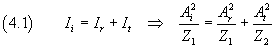Furthermore, we assume that the amplitude at the points of the surface is continuous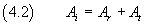So we have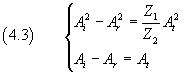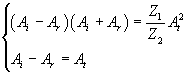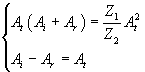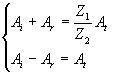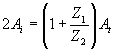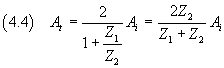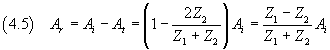The equations (4.4) e (4.5) show that:

• if the impedances are equal, the transmitted amplitude coincides with the amplitude of the incident amplitude and the reflected amplitude is zero;
• if Z1 is much greater than Z2, the transmitted amplitude tends to 0 and the reflected amplitude tends to coincide with the incident amplitude;
• if Z1 is much smaller than Z2, the transmitted amplitude tends to twice the incident amplitude and the reflected amplitude tends to coincide with the incident amplitude.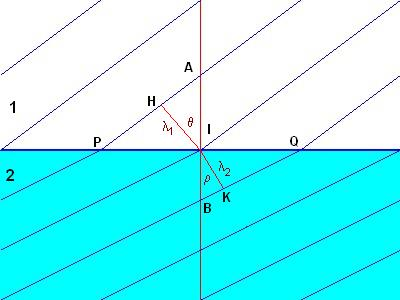In the figure, the black parallel lines in the half-plane 1 (white background) represent the wavefronts of a plane wave with velocity v1 and wavelength λ1 which crosses the surface of separation between the medium 1 and the medium 2 (blue background) in which the wavefronts are represented by blue parallel lines, the velocity v2 has magnitude less than that of v1 and the wavelength λ2 is less than λ1.
The line AB is perpendicular to the surface of separation and the segments HI and IK are perpendicular to the wavefronts.

Since the wavefronts are continuous, the segments are PI and IQ are equal. If we denote the angle HIA by θ and the angle BIK by ρ, we have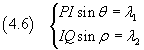Dividing side by side the equations (4.6) we have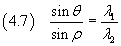Sinceλ=vT and the period T is the same in the two media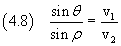The ratio between the speeds is a specific constant of the pair of two media; it is called the refractive index of the first medium with respect to the second one and is usually written n1,2.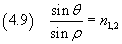The equation (4.9) is known as Snell's law or Descartes' law.

animation